Monday 08th August 2022CBSE Guess > Papers > Important Questions > Class XI > 2010 > Maths > Maths By Mr. Anil Kumar Tondak CBSE CLASS XI
Complex Nos. & Quadratic Equations Q.1. Show that: (a) 1 + i2 + i4 + i6 = 0 (b) ( 1 + i)8 ( 1 + 1/i)8 = 256 Q.2. Prove that: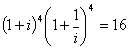Q.3. Simplify: ( a)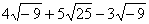( b)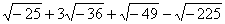Q.4. Express each one of the following in the standard form a + ib. (i)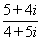(ii)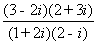(iii)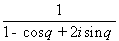Q.5. Express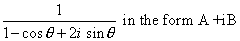Q.6. Represent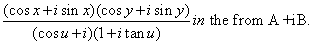Q.7. Express the following expression in the form of (a + ib):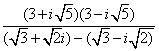Q.8. Prove that the following complex numbers are purely real: (i)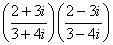(ii)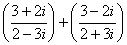Q.9. Reduce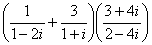to the standard form. Q.10. Express the following complex numbers in the standard form. Also, find their conjugate: (i)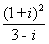(ii)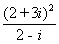Q.11. Find the conjugates of the following complex numbers: (i)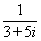(ii)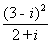(iii)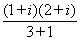Q.12. Write the following complex numbers in the polar form: (i) -3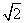+ 3i (ii) -1 – i Q.13. Find the modulus and principal argument of – 2 i. Q.14. Find the modulus of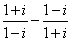. Q.15. Find the modulus and argument of the following complex numbers and hence express each of them in the polar form: (i)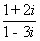(ii)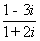Q.16. Find conjugate and modulus of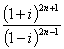Q.17. Find the conjugate of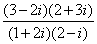Q.18. Find the conjugate of (3 - 2t) (2 + 3t) / (1 + 2t ) (2 - t) Q.19. Show that if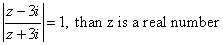Paper By Mr. Anil Kumar Tondak Email Id : [email protected] Ph No.: 9811363962